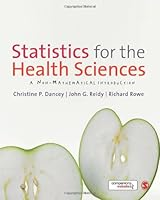Statistics for the Health Sciences: A Non-Mathematical IntroductionBook Description

This is a highly accessible textbook on understanding for the sciences, both conceptually and via SPSS. The authors give clear explanations of the concepts underlying statistical analyzes and descriptions of how these analyzes are applied in sciences research without complex statistical formulae. The book takes students from the basics of research , hypothesis , and descriptive statistical techniques through to more advanced inferential statistical tests that health sciences students are likely to encounter. Exercises and tips throughout the book allow students to practice using SPSS.